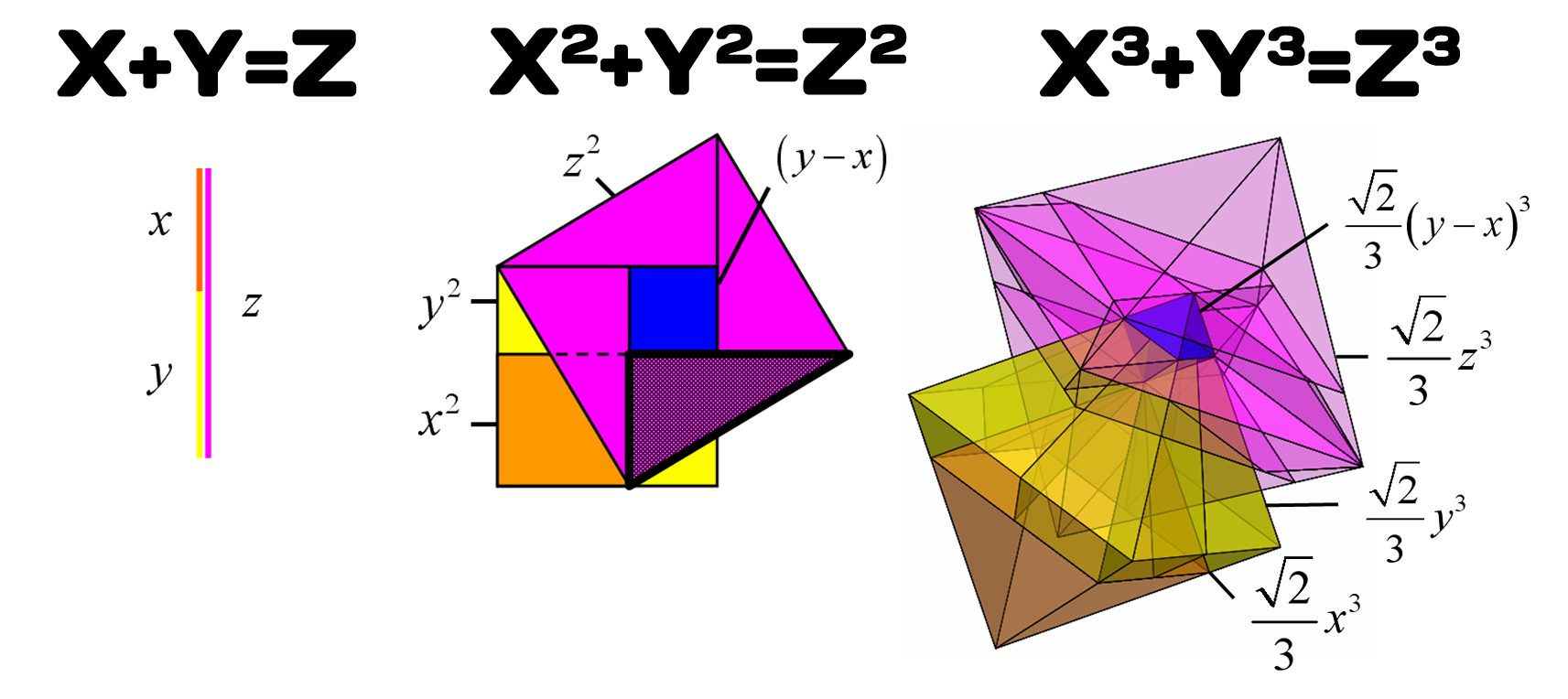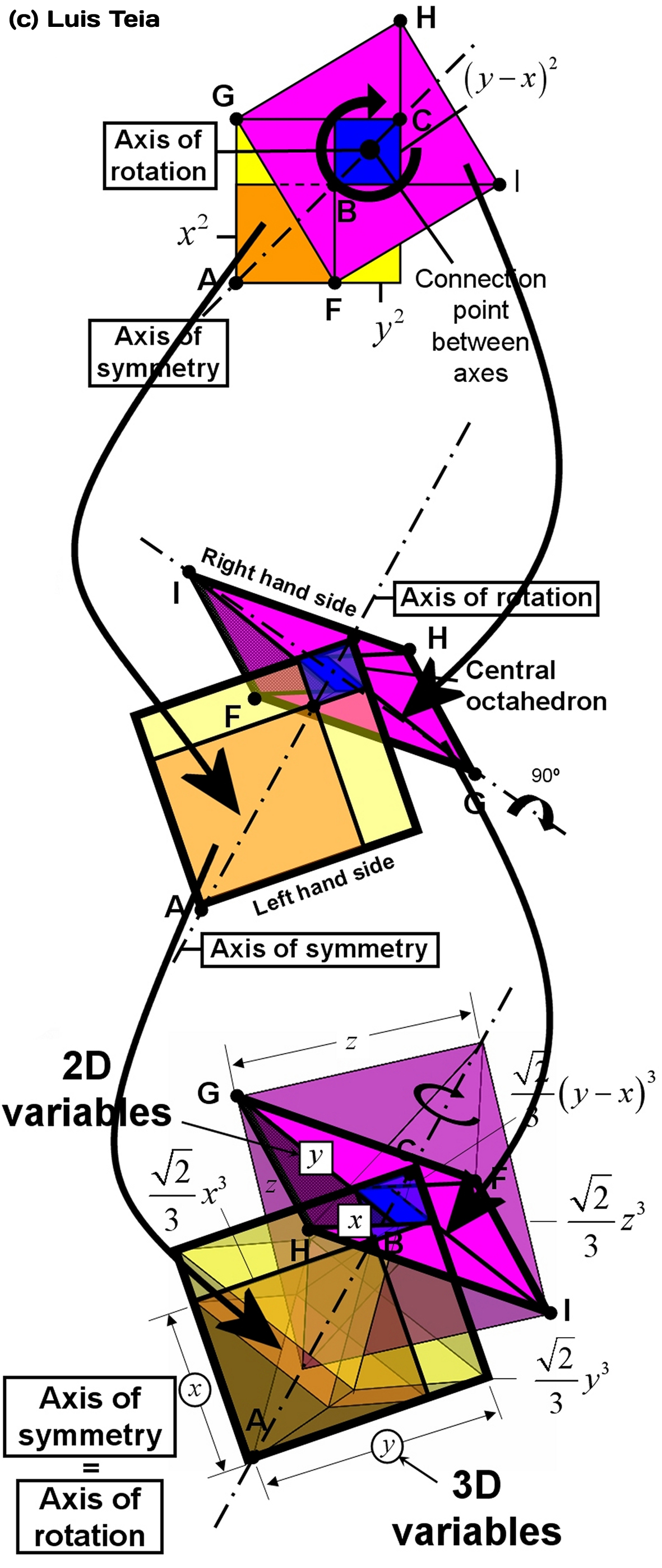# Revolution in the Pythagoras theorem?

•The Pythagoras’ theorem has changed. Better yet, our understanding of it has changed from two dimensions to three dimensions. For the past 2500 years, the Pythagoras’ theorem, arguably the most well-known theorem in the world, has greatly helped mankind to evolve. Its useful right angles are everywhere, whether it is a building, a table, a graph with axes, or the atomic structure of a crystal. It is universally applicable, but still, it is exclusively binding to two dimensions.[Image Source: Luis Gomes]

How does it look like in 3D? Until now, this was not known. For the past 5 years, Luis Teia has conducted a one-man quest to discover this mystery. Following from his recent paper, called Pythagoras triples explained via central squares published in the 2015 edition of the Australian Mathematics Senior Journal, he derived the proof. Let us briefly explain what this means.

While the 2D proof of the Pythagoras’ theorem is hand drawn and fits into half a page, the three-dimensional version requires a book with 120 pages. It is that complex! The geometrical part was drawn using computer-aided design (CAD), and the algebra of the mathematics spans 2/3 of the book. Remarkably, both the 2D and 3D Pythagoras’ theorems are interconnected. Moreover, the 3D is built from the 2D in a unique manner. This is shown below. The right-hand side of the theorem is rotated by 90 degrees. The blue square transforms into a blue octahedron – the three-dimensional equivalent of a square. This means that as the 2D theorem is built from squares, the 3D is built from octahedrons. Consequentially, one can find the 2D theorem inside the 3D by noting the presence of the shaded right-angle triangle. The implications of this can be taken a level deeper by realizing that the new 3D variables are governed by the 2D. Enough said! And, for the curious ones, the rest is explained in the book.

Most Popular

Why is this important? Considering the radical technological leap that Pythagoras imparted on mankind with his theorem some 2.5 millennia ago, the implications of this new 3D version can be equally significant, today. Perhaps, it leads to an “upgraded” three-dimensional version of our current trigonometry. Who knows? As for Teia’s next dream, he will be exploring the proof of Fermat’s theorem using this new approach.[Image Source: Luis Gomes]

Guest Author: Luis GomesSHOW COMMENT (1)